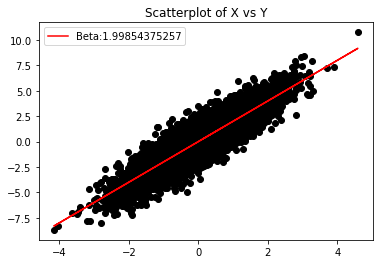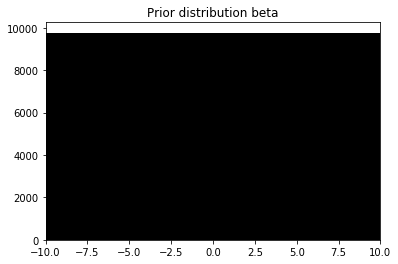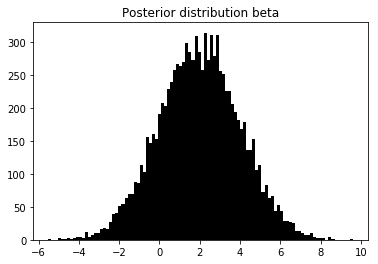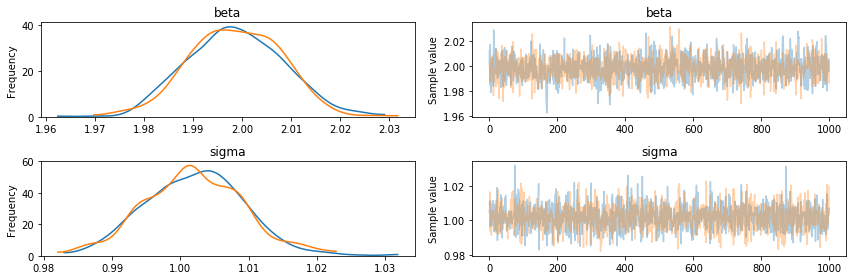# Bayesian linear regression¶

In this section we (briefly) develop the theory of Bayesian linear regression using scipy. If you are already familiar with the theory, I recommend having a look at PyMC3, PyStan or Edward for Bayesian modelling. In the last part we will motivate Bayesien regression with these tools, too.

As usual I do not take warranty for the correctness or completeness of this document.

In :
import scipy
from scipy import stats

%matplotlib inline
import matplotlib.pyplot as plt


When we infer the parameters of a linear regression model

\begin{align} \mathbf{y} \sim p(\mathbf{y} \mid \mathbf{X} ,\boldsymbol \beta, \sigma^2 ) = \mathcal{N}(\mathbf{X} \boldsymbol \beta, \sigma^2 \mathbf{I}_n), \end{align}

we usually compute the MLE $\hat{\boldsymbol \beta}$ as best solution of the problem. Here, $\mathbf{y}$ is a $n$-dimensional vector of (centered) Gaussian responses, $\mathbf{X}$ is a $(n \times p)$-dimensional design matrix, $\boldsymbol \beta$ is a vector of coefficients of appropriate size and $\sigma^2$ is a scalar.

In a Bayesian context, where we are (I would generally argue) primarily interested in analysis of beliefs, instead of establishing frequentist guarantees, we introduce prior information to the model (I took the term anaysis of beliefs from Larry Wasserman, I think).

As a refresher, the maximum likelihood estimate would be computed as:

\begin{align} \max_{\boldsymbol \beta, \sigma^2} \mathcal{L}(\boldsymbol \beta, \sigma^2) = & \max_{\boldsymbol \beta, \sigma^2} p(\mathbf{y} \mid \mathbf{X}, \boldsymbol \beta, \sigma^2),\\ =& \max_{\boldsymbol \beta, \sigma^2} \prod_i^n p(y_i \mid \mathbf{x}_i, \boldsymbol \beta, \sigma^2). \end{align}

Let's find the MLE for $\boldsymbol \beta$ on a random data set. First we generate some data.

In :
scipy.random.seed(23)

n = 10000
x = stats.norm.rvs(size=n)
beta = 2
y = x.dot(beta) + stats.norm.rvs(size=n)


We use scipy's polyfit function to get the MLE.

In :
fit = scipy.polyfit(x, y, 1)
fit_fn = scipy.poly1d(fit)

In :
plt.scatter(x, y, color="black")
plt.plot(x, fit_fn(x), color='red')
plt.legend(["Beta:" + str(fit)])
plt.title("Scatterplot of X vs Y")
plt.show()### Normal-Inverse Gamma Prior¶

In Bayesian linear regression, we put a prior distribution on the coeffients $\boldsymbol \beta$ and the variance $\sigma^2$.

Here, for the coefficients we will assume a Gaussian prior (as it is conjugate to a Gaussian likelihood for our responses $\mathbf{y}$) with mean $\mathbf{0}$ and variance $\sigma^2 \mathbf{I}$. For $\sigma^2$ we will use an inverse Gamma prior with hyperparameters $a$ and $b$.

\begin{align} \boldsymbol \beta \mid \sigma^2 &\sim \mathcal{N}(\mathbf{0}, \sigma^2 \mathbf{I}),\\ \sigma^2 &\sim \mathcal{IG}(a, b).\\ \end{align}

Other choices of priors are of course also fine. For demonstration this will do, though. I refer to Gelman's Bayesian Data Analysis for more information.

The joint prior distribution is given by:

\begin{align} \boldsymbol \beta, \sigma^2 &\sim \mathcal{NIG}(\mathbf{0}, \mathbf{I}, a, b),\\ p(\boldsymbol \beta, \sigma^2) &= p(\boldsymbol \beta \mid \sigma^2) \ p(\sigma^2) \\ &= \frac{1}{ (2\pi\sigma^2)^{\frac{p}{2}}} \exp\left( -\frac{1}{2\sigma^2} \boldsymbol \beta^T \boldsymbol \beta \right) \frac{b^a}{\Gamma(a)} \frac{1}{(\sigma^2)^{a+1}} \exp\left( -\frac{b}{\sigma^2} \right), \\ &\propto \frac{1}{(\sigma^2)^{\frac{p}{2} + a + 1 }} \exp \left( -\frac{1}{2\sigma^2} \boldsymbol \beta^T \boldsymbol \beta -\frac{b}{\sigma^2}\right) \end{align}

In the last part I removed every factor that does not depend on the variables $\boldsymbol \beta$ and $\sigma^2$.

Let's visualize these choices. First we draw $i \in \{1, \dots, 10000\}$ random samples $\sigma^2_i$ and then use these to sample coefficients $\boldsymbol \beta_i$. Both can be sampled directly using scipy, so we don't need to implement the random number generator.

In :
a = 1
b = 1
m = 0
var = 1

sigma_prior = stats.invgamma.rvs(size=n, a=a, scale=b)
beta_prior = scipy.zeros(n)
for i in range(n):
beta_prior[i] = stats.norm.rvs(size=1, loc=m, scale=sigma_prior[i] * var)

In :
beta_prior

Out:
array([  7.97751576,   1.56743705, -29.18229685, ...,   0.0530105 ,
-4.77214758,  -1.16903595])
In :
_, ax = plt.subplots()
ax.set_xlim(-10, 10)
ax.hist(beta_prior, bins=100, color="black")
plt.title("Prior distribution beta")
plt.show()Our prior for $\boldsymbol \beta$ looks uniform, even though it is technically Gaussian. Still, since it is conjugate, we can determine the posterior distribution analytically.

### Normal-Inverse Gamma Posterior¶

The posterior in a Bayesian model is proportional to the likelihood times the prior. As stated above, since the $\mathcal{NIG}$ prior is conjugate to the Gaussian likelihood, we will have a $\mathcal{NIG}$ posterior:

\begin{align} \text{posterior} &\propto \text{likelihood} \times \text{prior},\\ p(\boldsymbol \beta, \sigma^2 \mid \mathbf{X}, \mathbf{y}) \propto \; & p(\mathbf{y} \mid \mathbf{X}, \boldsymbol \beta, \sigma^2) \ p(\boldsymbol \beta , \sigma^2),\\ p(\boldsymbol \beta, \sigma^2 \mid \mathbf{X}, \mathbf{y}) \propto \; & \frac{1}{(\sigma^2)^{\frac{n}{2}}} \exp \left( -\frac{1}{2\sigma^2} (\mathbf{y} - \mathbf{X} \boldsymbol \beta)^T(\mathbf{y} - \mathbf{X} \boldsymbol \beta) \right)\\ & \; \frac{1}{(\sigma^2)^{\frac{p}{2} + a + 1 }} \exp \left( -\frac{1}{2\sigma^2} \boldsymbol \beta^T \boldsymbol \beta -\frac{b}{\sigma^2}\right),\\ = \; & \frac{1}{(\sigma^2)^{\frac{n}{2} + \frac{p}{2} + a + 1 }} \exp \left( -\frac{1}{2\sigma^2} (\mathbf{y} - \mathbf{X} \boldsymbol \beta)^T(\mathbf{y} - \mathbf{X} \boldsymbol \beta) -\frac{1}{2\sigma^2} \boldsymbol \beta^T \boldsymbol \beta -\frac{b}{\sigma^2} \right) \end{align}

As for the prior above, if we want to generate particles, we need to decompose $p(\boldsymbol \beta, \sigma^2 \mid \mathbf{X}, \mathbf{y})$ into $p(\boldsymbol \beta \mid \mathbf{X}, \mathbf{y}, \sigma^2) \ p(\sigma^2 \mid \mathbf{X}, \mathbf{y})$. The math is rather tedious, so I just give it here (adopted from Murphy's book). First we derive the new hyper parameter values $a$ and $b$ for the variance $\sigma^2$ as well as mean and variance for $\boldsymbol \beta$.

\begin{align*} p(\boldsymbol \beta, \sigma^2 \mid \mathbf{X}, \mathbf{y}) & = \mathcal{NIG}(\boldsymbol {\tilde{\mu}},\mathbf{\tilde{\Sigma}}, \tilde{a}, \tilde{b}),\\ \mathbf{\tilde{\Sigma}} &= (\mathbf{X}^T \mathbf{X} + \mathbf{I})^{-1},\\ \boldsymbol {\tilde{\mu}} &= \mathbf{\tilde{\Sigma}}\mathbf{X}^T \mathbf{y},\\ \tilde{a} &= a + \frac{n}{2},\\ \tilde{b} &= b + \frac{1}{2}(\mathbf{y}^T \mathbf{y} - \boldsymbol {\tilde{\mu}}^T \boldsymbol {\tilde{\Sigma}}^{-1} \boldsymbol {\tilde{\mu}}) \end{align*}
In :
var_tilde = 1 / (x.T.dot(x) + var)
m_tilde = var_tilde * x.T.dot(y)

a_tilde = a + n/2
b_tilde = b + .5 *( y.T.dot(y) - m_tilde * (1/var_tilde)* m_tilde)


Then we sample as above: first a particle from the posterior variance $p(\sigma^2 \mid \mathbf{y})$, then a particle from the conditional posterior $p(\boldsymbol \beta \mid \mathbf{y}, \sigma^2)$.

In :
sigma_post = stats.invgamma.rvs(size=n, a=a_tilde, scale=b_tilde)
beta_post = scipy.zeros(n)
for i in range(n):
beta_post[i] = stats.norm.rvs(size=1, loc=m_tilde, scale=sigma_post[i] * m_tilde)


Let's visualize this again:

In :
_, ax = plt.subplots()
ax.hist(beta_post, bins=100, color="black")
ax.lines
plt.title("Posterior distribution beta")
plt.show()## Probabilistic programming languages¶

It is rather unnessecary to implement these things yourself, especially for non-analytical solutions. For this tutorial we use PyMC3 to create the model, since we don't need Edward's tensorflow backend.

In :
import pymc3

In :
model = pymc3.Model()
with model:
sigma = pymc3.InverseGamma('sigma', 1, 1)
beta = pymc3.Normal("beta", mu=0, sd=sigma)
mu = x*beta
y_model = pymc3.Normal("Y", mu=mu, sd=sigma, observed=y)
model

Out:
$$\begin{array}{rcl} \text{beta} &\sim & \text{Normal}(\mathit{mu}=0,~\mathit{sd}=f(\text{sigma}))\\\text{sigma} &\sim & \text{InverseGamma}(\mathit{alpha}=1,~\mathit{beta}=1)\\\text{Y} &\sim & \text{Normal}(\mathit{mu}=f(array,~f(\text{beta})),~\mathit{sd}=f(\text{sigma})) \end{array}$$
In :
with model:
trace = pymc3.sample(1000)

Auto-assigning NUTS sampler...
Multiprocess sampling (2 chains in 2 jobs)
NUTS: [beta, sigma_log__]
100%|██████████| 1500/1500 [00:06<00:00, 226.49it/s]

In :
pymc3.traceplot(trace)
plt.show()In :
pymc3.summary(trace)

Out:
mean sd mc_error hpd_2.5 hpd_97.5 n_eff Rhat
beta 1.998542 0.009585 0.000245 1.980800 2.016965 1970.746687 0.999502
sigma 1.001999 0.007192 0.000153 0.988146 1.016780 2178.049637 0.999782